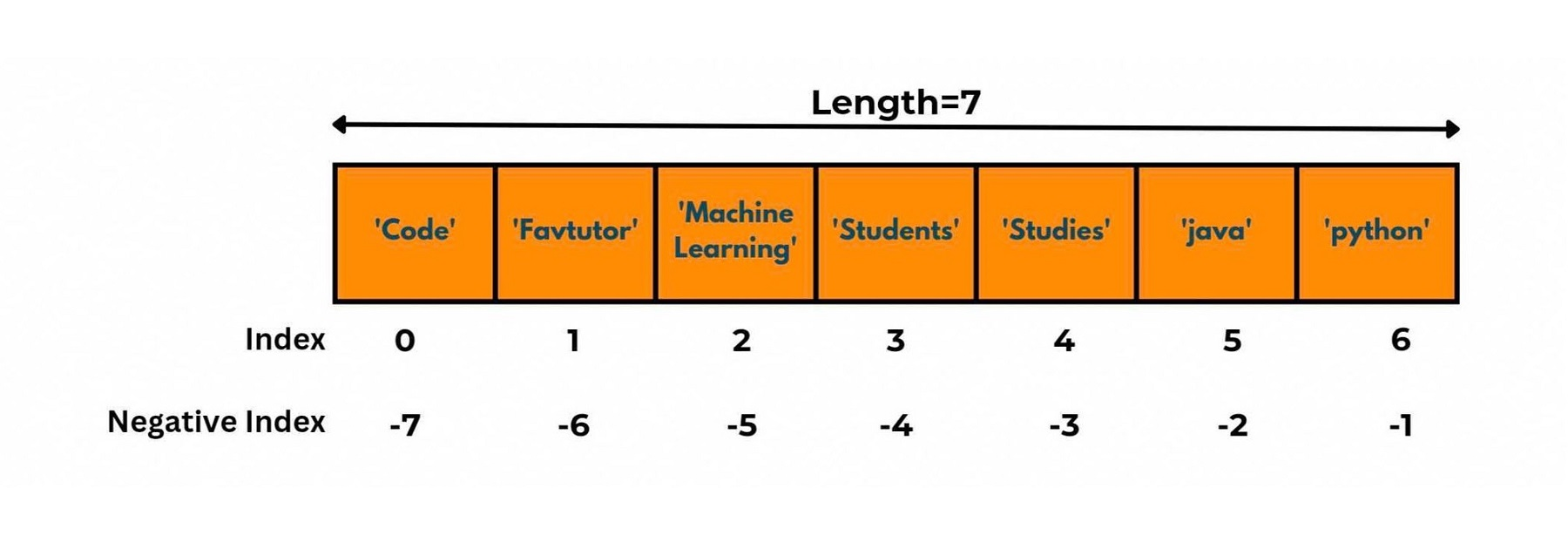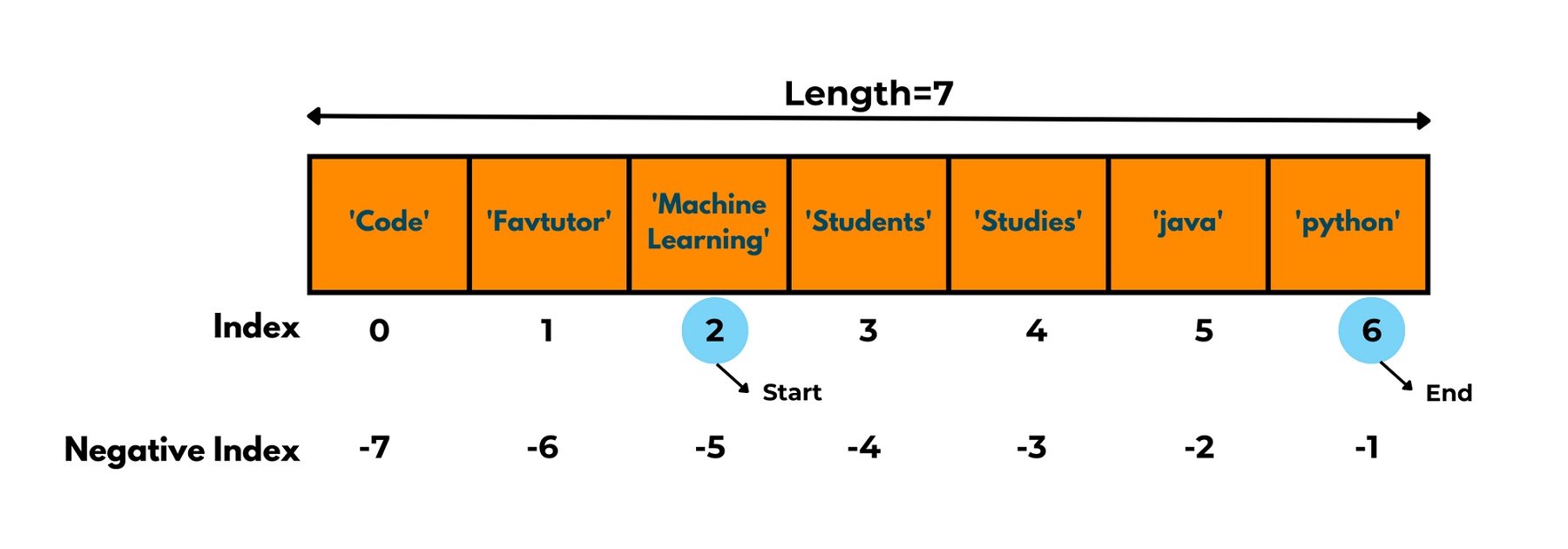# Python List index() & How to Find Index of an Item in a List?

One of the most adaptable data structures in python is lists. One of its key characteristics is the ability to use an index to find items on a list. In this article, we will learn how do you find the index of an item in a python list.

## What is a List Index in Python?

Each item in a list is assigned an index, which is a unique integer value that represents its position in the list. The first item in a list has an index of 0, the second item has an index of 1, and so on. It is a way to access an individual element in a list by its position or index number.

Keep in mind that list indexes always start from a 0 and go to the (n-1) value of elements. If the element that doesn't exist in the list using an index will result in an "IndexError".

Let's understand it with an example. We have the following list with seven values.As in the above example, the list has seven names stored in it but the index goes from 0 to 6.

Now to access elements of a list we need to call specific elements from a list by referring to Indexes. Here is an example of that:

```list=['Code', 'Favtutor', 'Machine Learning', 'Students', 'Studies', 'java', 'python']
print(list)
print(list)
```

Output:

```Code
Favtutor
```

In python, we use square brackets [ ] to call the elements of a list where inside the brackets we pass the index value of the location we want to read. It is our choice whether we can pass the positive index or negative index of an element; it will return the same desired output. For here if we call ‘list[-6]’ it will still return us the string ‘Favtutor’ as output.

## How to find the index of an item in a List?

Let's look at several methods to get the python index of a list item:

### 1) Index() method

The simplest method to find the index of an item is by using the built-in index function. The index() method returns the index of the first occurrence of the item in the list. It takes the value of the search element as the argument and gives us the index of where it appears the first time in the list.

Here is the simple syntax of the index method: list_name.index(‘item_name’).

Index() function can have a maximum of 3 arguments out of which 1 is a mandatory argument and the rest can be optional:

• Element: Item name you want to search
• Start: Starting index to start the search for the element.
• End: Ending index to stop the search of the element after this index.

Look at the below code:

```list=['Code', 'Favtutor', 'Machine Learning', 'Students', 'Studies', 'java', 'python']
print("index of Studies is",list.index('Studies'))
print("index of java is",list.index('java',2,6))
```

Output:

```index of Studies is 4
index of java is 5
```

The image will better illustrate this:As you can see, we attempt to print the index at which a specific element is present. This is equivalent to locating the memory address for that value. In the initial printing statement, we only give the element name.

The Index() function then searches the entire list for the element and returns the index id if it is present. But, we have set the values of two optional parameters of the Index() function in the second printing statement, which essentially slices the list by that index and searches for the element in the new sliced list only, returning the index if it exists otherwise raising a ValueError.

### 2) Enumerate function

We can also use an enumeration for getting the index of a list item. It is a built-in python function that is similar to Index() function.

The only difference between them is that the Enumerate() function can return all the index positions at which a specific element is present, as opposed to Index(), which only returns the index of an element's first occurring position.

Here is an example of this function:

```list=['Code', 'Favtutor', 'java','Machine Learning', 'Students', 'Studies', 'java', 'python','java']
index = [i for i ,e in enumerate(list) if e == 'java']
print("Index for Value java: ", index)
```

Output:

`Index for Value java:  [2, 6, 8]`

### 3) Using Numpy module

The Numpy library also allows us to find an index of any element in a list using its pre-built function np.where() but for that, you need to first convert your list into a numpy array using np.array() function. It also returns all indexes of occurrence for that element.

Here is the code for this function:

```import numpy as np
list=['Code', 'Favtutor', 'java','Machine Learning', 'Students', 'Studies', 'java', 'python','java']
array=np.array(list)
index= np.where(array=='java')
print("Index for Value java: ", index)
```

Output:

`Index for Value Students:  (array([2, 6, 8], dtype=int64),)`

## Conclusion

You learned about the Python index in this article and looked at some practical examples to get the index of an element in a list. Now try exploring more programs yourself. Happy Learning :)

### FavTutor - 24x7 Live Coding Help from Expert Tutors!